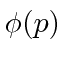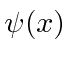## Wave Packets and Uncertainty

The probability amplitude for a free particle with momentumand energyis the complex wave functionNote thateverywhere so this does not represent a localized particle. In fact we recognize the wave property that, to have exactly one frequency, a wave must be spread out over space.

We can build up localized wave packets that represent single particles by adding up these free particle wave functions (with some coefficients).(We have moved to one dimension for simplicity.) Similarly we can compute the coefficient for each momentumThese coefficients,, are actually the state function of the particle in momentum space. We can describe the state of a particle either in position space withor in momentum space with. We can useto compute the probability distribution function for momentum.We will show that wave packets like these behave correctly in the classical limit, vindicating the choice we made for.

The Heisenberg Uncertainty Principle is a property of waves that we can deduce from our study of localized wave packets.It shows that due to the wave nature of particles, we cannot localize a particle into a small volume without increasing its energy. For example, we can estimate the ground state energy (and the size of) a Hydrogen atom very well from the uncertainty principle.

The next step in building up Quantum Mechanics is to determine how a wave function develops with time - particularly useful if a potential is applied. The differential equation which wave functions must satisfy is called the Schrödinger Equation.

Jim Branson 2013-04-22